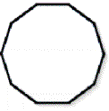HOME MATH DICTIONARY DOWNLOAD FEEDBACK DISCLAIMER
 Question: What is a Decagon ? Answer: A Decagon is a polygon with 10 sides. When all of its sides and angles are equal it is said to be regular. In geometry, a decagon is any polygon with ten sides and ten angles, and usually refers to a regular decagon, having all sides of equal length and all angles equal to 144°, therefore making each angle of a regular decagon be 144°. Example of a Decagon: